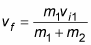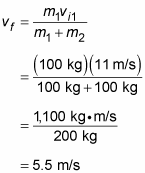##### Physics I For Dummies, 3rd EditionYou can use the principle of conservation of momentum to measure characteristics of motion such as velocity. Say, for example, that you’re out on a physics expedition and you happen to pass by a frozen lake where a hockey game is taking place. You measure the speed of one player as 11.0 meters per second just as he collides, rather brutally for a pick-up game, with another player initially at rest. You watch with interest, wondering how fast the resulting mass of hockey players will slide across the ice.

After asking a few friends in attendance, you find out that the first player has a mass of 100 kilograms and the bulldozed player (who turns out to be his twin) also has a mass of 100 kilograms. So what’s the final speed of the player tangle?

You’re dealing with a closed system, because you neglect the force of friction here, and although the players are exerting a force downward on the ice, the normal force is exerting an equal and opposite force on them; therefore, the vertical force sums to zero.

But what about the resulting horizontal speed along the ice? Due to the principle of conservation of momentum, you know that

pf = pi

Imagine that the collision is head on, so all the motion occurs in one dimension — along a line. So you only need to examine the components of the vector quantities in this single dimension. The component of a vector in one dimension is just a number, so you don’t write them in bold.

The victim isn’t moving before the hit, so he starts without any momentum. Therefore, the initial momentum, pi, is simply the initial momentum of the enforcer, Player 1. To put this equation into more helpful terms, substitute Player 1’s mass and initial velocity (m1vi1) for the initial momentum (pi):

pi = m1vi1

After the hit, the players tangle up and move with the same final velocity. Therefore, the final momentum, pf, must equal the combined mass of the two players multiplied by their final velocity, (m1 + m2)vf, which gives you the following equation:

(m1 + m2)vf = m1vi1

Solving for vf gives you the equation for their final velocity:Plugging in the numbers gives you the answer:The speed of the two players together will be half the speed of the original player. That may be what you expected, because you end up with twice the moving mass as before; because momentum is conserved, you end up with half the speed. Beautiful. You note the results down on your clipboard.